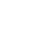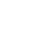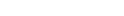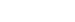# Odds Converter

The simplest sports betting math many punters possess is the ability to convert odds and calculate break even percentages, also known as implied probabilities. After spending years doing these calculations, first on a windows calculator, and later in an excel spread sheet, I finally developed the Online Gambling odds converter you’ll find below.

## Odds Converter Tool

[oddscalc]

In case you’re not familiar with the difference between American Odds called moneylines and European odds, which are in decimal format, here is how each works.

## American Odds

Also known as moneylines, are offered as either a positive or negative number. When positive, the moneyline represents how much profit a punter stands to make on a \$100 stake. To give an example, a line of +125 represents risk \$100 to win \$125 profit. Negative moneylines represent how much a punter needs to stake for a winning bet to profit \$100. A moneyline of -235 is risk \$235 to win \$100 profit.

## European Odds

Also known as decimal odds, represent how much a winning ticket is worth for each dollar staked. For example if a punter wagers \$10.00 at odds 1.95 and wins, their payout is \$10 x 1.95 = \$19.50. A major difference between American and European odds is that while the American version is based on profit, the European version is based on return. Likewise, a \$10 wager on odds 1.95 translates to risk \$10.00 to potentially profit \$9.50. Considering 1.95 pays less than even money, it would be expressed in American odds format as a negative moneyline.

The best way to convert American odds to European format, and vice versa, is to use the “odds converter” located above. For those of you interested in the math, I’ll cover it great detail later in this article. First let me discuss break even percentage and implied probability.

## Implied Probability

Is how often a bet must win for it to have neutral value. To give the simplest example, take American odds +100 (which is the equivalent of 2.00 in European odds) where winning bets are paid even money, here the implied probability is 50%. This is because in American format risk \$100 to win \$100, and in European format stake \$1 to return \$2, you’ll need to win an average of 50% of the time to break even over the long haul.

Where our odds converter comes in handy is let’s say you’ve done an analysis on a game between the Boston Red Sox and Tampa Bay Rays and determined the Red Sox should be 57% favorites in this game. After price shopping, the best odds you can find on the Red Sox are -128, which in European format is 1.781. There are now two ways you can determine if you’ve found a good bet. The first method is to plug 57% into our odds converter under the required break even field. Doing this you’ll find at 57% the true odds in American format are -132.6 (1.754 European). Consider you’re getting better than this with the -128 (1.781 European) you have a profitable bet.

The second way you could approach the above problem, is to plug -128 into our odds converted under American odds. Here you’ll see the required break even rate (implied probability) is 56.14%. Considering your calculation of a 57% win probability comes out higher than the win rate needed to break even, you’ve find yourself a good bet.

## Sports Betting Strategy You Should, but Most Don’t, Understand

For those with basic sports betting math skills, the sample problem I’m about to give will be easy to solve. If you’re stumped, or answer incorrectly, what you’re about to read could very well be what gets you on the path required to winning consistently at sports betting.

### The sample

Every online betting site with odds on a NBA basketball game between the Orlando Magic and Sacramento Kings has the odds listed in American format as Magic -545 / Kings +445.

Someone comes long and says “I’ll offer you either Magic -490 or Kings +490, your choice”. Which of these options, if any, has the highest expected value?

The way many sports bettors would approach this is to average out 545 and 445 and figure the fair value is about -495 / +495. Using this failed logic they’d end up taking the Magic -490 as that’s better than the fair price and must be +EV. The problem is neither American nor European odds work this way; they can’t be averaged out to calculate the fair value.

The first step those who understand sports betting math take to solve this problem is the conversion of Magic -545 / Kings +445 to break even percentages.

After plugging the odds into our odds converted I find:

• Magic -545 = 84.50% required break even percentage
• Kings +445 = 18.35% required break even percentage.
• Adding these together you’ll find they total 102.85%. They total more than 100% because juice, which is how the bookmakers profit, is included in the -545/+445 lines. To remove juice we divide each by 102.85%.
• Magic -545 = 84.50%/102.85% = 82.16%
• Kings +445 = 18.35%/102.85% = 17.84%

To check double check our math 82.16 + 17.84% = 100%. We’ve successfully removed the juice and now have each team’s no-vig win probability.

Next go back to our odds converter and plug in the no vig win probabilities into the field titled “required break even %. Doing this we see:

• Magic at 82.16% = -460.5 in American odds format.
• Kings at 17.84% = +460.5 in American odds format.

The offer we were given was Magic -490 or Kings +490 and we calculated the fair prices with no vig are Magic -460.5 and Kings +460.5. The obvious best bet from +EV/-EV standpoint here is Kings +460.5.

The beautiful thing about sports betting, there are dozens of sites each with unique lines on the same game. If you’re able to gauge the consensus line by looking at what the discount bookmakers and exchanges such as Pinnacle, 5Dimes, and Betfair are offering, when you spot a line that is off at a recreational betting site such as Sportsbook.com, Bodog, or Intertops, you’ll have no problem calculating which side if any is a profitable long term bet. The ability to do this alone, combined with bonuses and promotions is enough for the average sports bettor to make some serious extra coin each season. With that said, be sure to bookmark this page so you have quick access to our odds converter as it’s a tool serious sports bettors will want to use often.

## Math to Convert Betting Odds

To conclude this article I’ll share the math involved in converting odds and break even percentages. Remember our odds converter can do this math for you at the click of a button.

### Converting American odds to European Odds

The formula to convert American moneylines to European decimals odds depends if the moneyline is expressed using a positive or negative number.

#### Positive Numbers

To convert a positive moneyline to European decimal odds take the moneyline, divide it by 100 and then add 1. The formula is (Moneyline/100) +1. To give an example, the math to convert +358 is (358/100) +1 which calculates to European odds of 4.580.

#### Negative Numbers

To convert a negative moneyline to European decimal odds take 100 divide it by the moneyline (without using the negative sign) and then add one. The formula is (100/Moneyline) + 1. To give an example, the math to convert -115 is (100/115) +1 which calculates to European odds of 1.870.

### Converting European Odds to American Odds

The formula to convert European odds (decimals) to American moneyline format depends if the odds are 2.00+, or 1.999 or less.

#### Odds \$2.00+

To convert European decimal odds 2.00 or greater to an American moneyline the math is: 100*(Decimal odds -1). For example, odds 2.183 use the formula 100*(2.183-1) which calculates to an American moneyline of +118.3.

#### Odds Under \$2.00

To convert European decimal odds less than 2.000 to an American moneyline the math is (-100)/(Decimal odds – 1). For example, odds 1.71 uses the formula -100/(1.71 -1) which calculates to an American moneyline of -140.8.

## Calculating Break Even Percentage

When dealing with European decimal odds, calculating how often a bets must win to break even is simple division. The formula is (1/odds=%). To use decimals odds 2.15 as an example, the math is 1/2.15 which equals 0.4651; converted to a percentage this is 46.51%. That’s how often a bet at 2.15 must win to have neutral value and is referred to as its break even rate or implied probability.

When dealing with American odds the formula to calculate a bets required break even rate (implied probability) is risk/return=implied probability, where return is how much a winning ticket would pay. For example at -215 a sports bettor wagers \$215 to win \$100, a winning bet returns \$315 (the \$215 stake and \$100 win). In this case the math to calculate implied probability is 215/315 which equals 0.6825 and as an implied probability is 68.25%.

## Converting Win Probabilities to Fair Odds

In cases where you know a team is going to win a certain percentage of the time and you’re looking to convert this percentage into fair European decimal odds or an American moneyline, this can be done with simple math. First change your percentage to a decimal (example 50.5% is 0.505) then use the formula (1/probability=European Odds). In the case of 50.5% the math is 1/.505 which equals European decimal odds of 1.98. To convert this to American odds go back to our formula (-100)/(Decimal odds – 1) which calculates to a moneyline of -102. As a reminder, in cases where the decimal odds are 2.0 or greater the moneyline will come out a positive number and therefore the conversion formula is 100*(Decimal odds -1) instead of the (-100)/(Decimal odds – 1) used in this example.

## Best Online Betting Odds

Now that you understand how to calculate odds, you’ll likely appreciate how impressive the odds offered at www.5dimes.eu are. While no doubt the most popular reduced juice option for American players, 5Dimes also offers soccer, cricket, Euro hockey, Rugby League, Rugby Union, Aussie Rules, volleyball, motor racing, golf, tennis, snooker, water polo and darts at better odds than High Street bookmakers and TAB. To learn more read our 5Dimes Review.

Of course, the best advice we can give you at Online Gambling is to keep learning sports betting math, while using dozens of accounts to shop lines. We hope you enjoyed this article on converting odds, and wish you the best of luck this sports betting season.